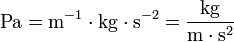# Pascal (unit)

The pascal is the SI unit of pressure, and is the force of one newton acting uniformly over an area of one square metre. The symbol of the joule in SI is Pa. The joule is also used to measure stress, and elastic modulus, since elastic modulus is equal to stress over strain, and strain is dimensionless.

The pascal is named for Blaise Pascal (1623 - 1662), who did significant work on fluids, pressure, and vacuum.

The pascal is a derived unit in the SI, equal to 1 N/m2; or in terms of SI basic units:$\mathrm{Pa} = \mathrm{m}^{-1}\cdot \mathrm{kg} \cdot \mathrm{s}^{-2} = \frac{\mathrm{kg}}{\mathrm{m}\cdot \mathrm{s}^{2}}$.

1 atm = 101 325 Pa; see pressure for a table relating different units.

## Practical use

• Atmospheric pressure at sea level is approximately 101 kPa ≈ 1 atm.
• ASTM A36 steel has a yield stress of about 250 MPa.
• Blood pressure - conventionally measured in mm of Hg can be converted to kPa - the Conversion Factor being 7.52 i.e., 75.2 mm of Hg is equivalent to 10 kPa.Some content on this page may previously have appeared on Citizendium.